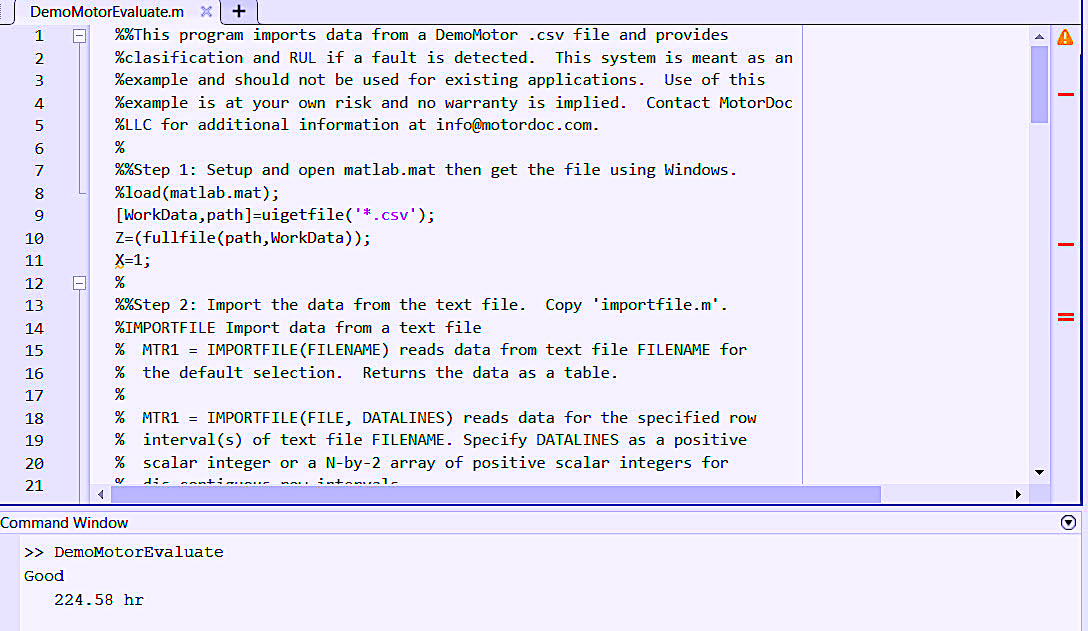512-800-6031 editor@ramreview.com

This week’s article concludes our seven-part series. In Parts V and VI (see links below), we developed the classification and Remaining Useful Life (RUL) models that we would use in our Machine Learning project. The final step in developing our tool is to convert it to a code, or script, that allows us to obtain useful information. This requires a degree of organization, error-handling, and testing, at which point some rework might be required.

The first thing we want to do in our software development is import data for analysis. For individual datasets and testing, we can bring in a *.csv (comma delimited file), verify it, and run it through the different processes that we’ve developed. At the end of the testing phase, it’s possible to rewrite the first part to gather information from a data historian or other means of data collection.

For this part of our project, since we’re working with MatLab, the function for importing files into the software can be cut and pasted into the script. MatLab’s “uigetfile” function can be used to open a file-selection dialog. The error handlers within the original import function will handle most conditions with additional coding that we add during testing.  Figure 1 represents the beginning of the code. (NOTE: A link to this code can be found in the Sidebar at the end of this article.)Fig. 1. MatLab code for motor evaluation with classification and RUL results.

The next step is to “smooth” the data and eliminate outliers. For our purposes, we’ll select a simple “movmedian” MatLab function using a window of five datasets (rows or hours). We will also select the specific data that we want to include in smoothing. Variables such as time would be excluded, as that should be in fixed increments. We will also separate out the last row of data to check if the motor is running, which can be accomplished by looking at data such as speed or voltage. For our project, we will be using speed so that, as we add additional types of defects to the software, the loss of a phase or single phasing does not present as a motor not running.

If the motor is operating, we would then process the same single line of data through our classification model that was developed in Part V (Sept. 5, 2021) of this article series. The result would produce the same output, or finding, as was used in the “FaultCode” variable. As we used a numerical value, we would then convert it to a text output which can be presented after the data is processed.

The RUL should be processed separately because, in some cases, a fault may not show exactly as identified in training RUL prediction function. (This approach provides a second layer of detection.)  In this case we will limit the time to failure detection to 800 hours in code as we run each trained RUL prediction function.

Following some experimentation after the steps described in our Part VI article (Sept. 12, 2021), in which we test the options between linear and exponential degradation, we selected linear degradation for all three measurements in voltage unbalance (Vu), current unbalance(Au), and power factor (PF). We then selected the lowest value of RUL and, if under 800 hours, present it as an output.

SERIES WRAP-UP

The purpose of this series has been to demonstrate the process surrounding a simple machine learning project. Over the course of seven articles, we have identified a need, evaluated and created data sets, identified the need to pre-process the data, created classification and RUL algorithms, and, as shown this week, brought the system together into a useable code. In the process, we used one of many tools that are available for this type of work, such as MatLab and Python. Other commercial systems are available for intranet and cloud-based systems.TRR

SIDEBAR: Sample Code for Motor Evaluation with Classification and RUL Results
For those wishing to explore the method described in this article (see Fig. 1), Mathworks provides 30-day trials of the personal and business versions of MatLab and Simulink. The code created for this ML-article series was developed within MatLab. The resulting code (or script) can be downloaded in the following link:

Part I  (Aug. 8, 2021)

Part II (Aug. 15, 2021)

Part III (Aug. 21, 2021)

Part IV (Aug. 30, 2021)

Part V (Sept. 5, 2021)

Part VI (Sept. 12, 2021)